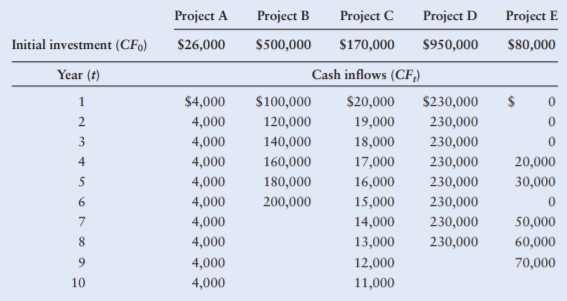### Create an Account

Already have account?

### Forgot Your Password ?

Home / Questions / Net present value Independent projects Using a 14 cost of capital calculate the net presen...

# Net present value Independent projects Using a 14 cost of capital calculate the net present value for each of the independent projects shown in the following table and indicate whether each is

Net present value—Independent projects Using a 14% cost of capital, calculate the net present value for each of the independent projects shown in the following table, and indicate whether each is acceptable.Jun 03 2021 View more View Less

#### Answer (UnSolved)Get Solution